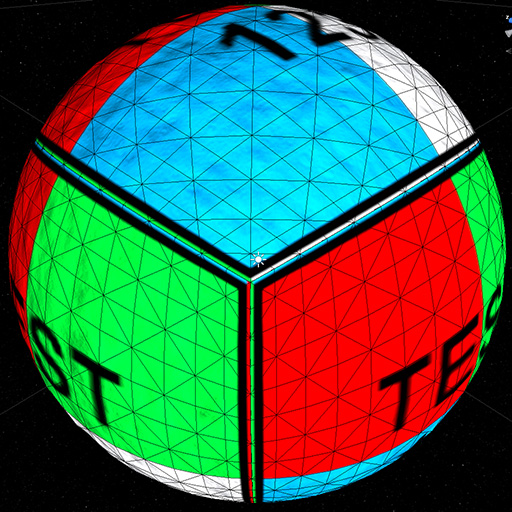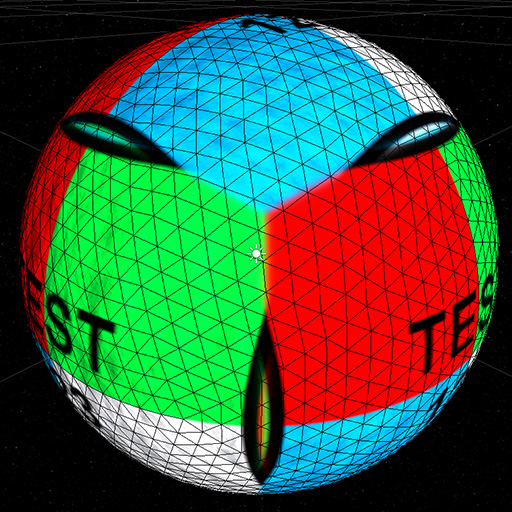###Author Topic: Mar 1, 2016 - Terrain Generation (Part 2)  (Read 2283 times)

#### ArenMook

• Hero Member
•• Thank You
• -Given: 337
• Posts: 22,154##### Mar 1, 2016 - Terrain Generation (Part 2)
« on: March 01, 2016, 08:37:23 AM »
In the previous post I mentioned that I decided to scrap using Unity's terrain system in favor of a home brew solution, and now I'm going to explain why.

First, let's examine what the Unity system can do. It can accept a heightmap and it expects splat maps (basically textures) for any additional information you need to pass to it. It handles LOD for you silently and with minimal control over it, and the terrains are expected to be flat (that is, they can't curve from edge to edge). The terrains it generates are are always rectangular or square. Still, as show in the previous post even with these limitations it's possible to create interesting looking terrains. The fairly low draw far clipping plane gives the illusion of curvature where there isn't one:Regardless, what I'd like to have, is a terrain system on a planetary scale. While I don't necessarily want the ability to go from orbit to the planet's surface, it would be nice to have the tech that can do that -- simply because it would be cool. The terrain I'd like would need to be curved to make it possible to create round planets, and travel from any point on the planet to any other point without loading times, given enough patience and a fast enough vehicle. I can, in theory, just fill the terrain data in such a way that I can still use rectangular terrains to circumnavigate the planet (think a rectangle that simply gets projected onto a sphere that I can move around freely)... but what about supporting smaller celestial bodies such as large asteroids with a diameter of a few hundred meters? The curvature would need to be very evident in that case, and it would be more difficult to pull it off with the existing terrain. Doable though -- can just write a shader that adjusts the vertex positions as they get farther from the player.

In any case, thinking about all of this made me consider other possibilities, such as generating my own planetary terrain. Taking a quick glance on the Asset Store revealed two packages that could help. Unfortunately neither of them panned out for different reasons ranging from flat compile errors and naive approaches (10,000 diameter sphere? ouch!) to the vertex position code being hidden somewhere where I couldn't even find it. Next step? Playing around with it myself, of course.

First, how does one generate a spherical planet and deform it? Well, the LibNoise noise generation is already 3-D by default, so it's really a simple matter of generating a sphere. There are 3 common approaches of generating a sphere. The most common is a regular sphere one can create using the GameObject menu in Unity. It looks like this:The most obvious problem with this sphere is the vastly uneven triangle sizes and difficulty in sub-dividing them to create a more dense mesh. The latter is necessary in order to improve details around the camera. So... that's out.

The second approach is to start with a cube made up of 6 planes like so:... then simply normalize the position of every vertex, thus creating a sphere:That's better! Subdividing each face is a trivial matter of splitting it into 4 child faces, so it would be trivial to improve the level of detail of the terrain closer to the camera. Additionally, since each side is technically still a square, so putting textures on it is a trivial matter. There is just one small problem... the sizes of the triangles can vary by quite a bit, depending on whether the triangle is close to the center or the corners of the mesh. Take this picture for example, taken from the same distance to the sphere's surface, just at two different points:As you can see, not only the sizes of the triangles are vastly different, but the regions that are square on the left actually look like skewed rectangles on the right. If the player is standing at the intersection point on the terrain that's on the right hand side, he would be surrounded by 3 very skewed regions! While not a total deal breaker, it's still something that would be nice to avoid, if possible.

Enter solution #3: icosahedron. The best thing about using icosahedrons is that they're made up of equilateral triangles -- that is perfect triangles with all 3 sides of completely equal length. This means that every triangle is going to be the same exact size as any other. No skewing of any kind! Subdividing each triangle is a trivial matter as well -- simply split each one into 4 by dividing each line connecting points right down the middle. There's just one problem... it's not possible to use the same kind of texture mapping for an icosahedron that can be used for other shapes as there are no squares to work with:So how would one do texture mapping? Well... while it's true that using vertex texture coordinates may be a problem, one could still simply use the normalized position of the vertex to figure out where that resulting vector projects onto the 6-sided cube, thus resulting in usable UV coordinates. This kind of approach is commonly known as tri-planar mapping:Like with the terrain options before it I also checked the Asset Store offerings... unfortunately doing tri-planar mapping properly involves sampling each side of the cube independently, or normal mapping doesn't work properly and you end up with pixels that get lit even though they are supposed to be shadowed and vice versa. All the Asset Store offerings for Unity 4 suffered from this issue -- even my old deprecated Space Game Starter Kit tri-planar asteroid shader, amusingly enough. Also, to do tri-planar mapping properly, it's important to ensure that no two adjacent surfaces have tangents pointing in opposite directions, or visible rendering artifacts can appear on those edges (especially when specular lighting is used). So... I had to create a new one that does what I wanted:The shader itself is below in case you need it.

1. Shader "SW/Triplanar Bumped"
2. {
3.         Properties
4.         {
5.                 _Color ("Main Color", Color) = (1,1,1,1)
6.                 _MainTex ("Base (RGB)", 2D) = "white" {}
7.                 _BumpMap ("Normal Map", 2D) = "bump" {}
8.                 _BlendPower ("Blend Power", Float) = 64.0
9.                 _SpecColor ("Specular Color", Color) = (0.5, 0.5, 0.5, 1)
10.                 _Shininess ("Shininess", Range (0.03, 1)) = 0.078125
11.         }
12.
14.         {
15.                 LOD 300
16.                 Tags { "RenderType" = "Opaque" }
17.
18. CGPROGRAM
19. #pragma target 3.0
20. #pragma surface surf Proper vertex:vert
21.
22. sampler2D _MainTex;
23. sampler2D _BumpMap;
24. half4 _MainTex_ST;
25. half4 _Color;
26. half _BlendPower;
27. half _Shininess;
28.
29. struct Input
30. {
31.         float3 worldPos;
32.         float3 normal;
33. };
34.
35. half4 LightingProper (SurfaceOutput s, half3 lightDir, half3 viewDir, half atten)
36. {
37.     half3 n = normalize(s.Normal);
38.     half3 h = normalize(lightDir + viewDir);
39.     half diff = max(0.0, dot (n, lightDir));
40.     half nh = max(0.0, dot (n, h));
41.     half spec = pow(nh, s.Specular * 128.0) * s.Gloss;
42.
43.     half4 c;
44.     c.rgb = (s.Albedo * _LightColor0.rgb * diff + _LightColor0.rgb * _SpecColor.rgb * spec) * atten;
45.     c.a = s.Alpha + _LightColor0.a * _SpecColor.a * spec * atten;
46.     return c;
47. }
48.
49. inline float3 GetContribution (float3 normal)
50. {
51.         half3 contribution = pow(abs(normalize(normal)), _BlendPower);
52.         return contribution / dot(contribution, 1.0);
53. }
54.
55. void vert (inout appdata_full v, out Input o)
56. {
57.         UNITY_INITIALIZE_OUTPUT(Input, o);
58.         o.normal = v.normal;
59.         half3 signage = sign(v.normal);
60.         half3 contribution = GetContribution(v.normal);
61.         v.tangent = half4(contribution.y, -signage.z * contribution.z, signage.x * contribution.x, -1.0);
62. }
63.
64. void surf (Input IN, inout SurfaceOutput o)
65. {
66.         half3 signage = sign(IN.normal) * 0.5 + 0.5;
67.         half3 contribution = GetContribution(IN.normal);
68.         half3 pos = mul(_World2Object, float4(IN.worldPos, 1.0)).xyz;
69.
70.         half2 tc0xy = half2(-pos.z,  pos.y) * _MainTex_ST.xy + _MainTex_ST.zw;
71.         half2 tc0zw = half2( pos.z,  pos.y) * _MainTex_ST.xy + _MainTex_ST.zw;
72.         half2 tc1xy = half2( pos.x, -pos.z) * _MainTex_ST.xy + _MainTex_ST.zw;
73.         half2 tc1zw = half2( pos.x,  pos.z) * _MainTex_ST.xy + _MainTex_ST.zw;
74.         half2 tc2xy = half2( pos.y, -pos.x) * _MainTex_ST.yx + _MainTex_ST.wz;
75.         half2 tc2zw = half2(-pos.y, -pos.x) * _MainTex_ST.yx + _MainTex_ST.wz;
76.
77.         half4 c =
78.                 lerp(tex2D(_MainTex, tc0xy), tex2D(_MainTex, tc0zw), signage.x) * contribution.x +
79.                 lerp(tex2D(_MainTex, tc1xy), tex2D(_MainTex, tc1zw), signage.y) * contribution.y +
80.                 lerp(tex2D(_MainTex, tc2xy), tex2D(_MainTex, tc2zw), signage.z) * contribution.z;
81.
82.         o.Albedo = c.rgb * _Color.rgb;
83.         o.Alpha = 1.0;
84.
85.         o.Normal = UnpackNormal(
86.                 lerp(tex2D(_BumpMap, tc0xy), tex2D(_BumpMap, tc0zw), signage.x) * contribution.x +
87.                 lerp(tex2D(_BumpMap, tc1xy), tex2D(_BumpMap, tc1zw), signage.y) * contribution.y +
88.                 lerp(tex2D(_BumpMap, tc2xy), tex2D(_BumpMap, tc2zw), signage.z) * contribution.z);
89.
90.         o.Gloss = c.a * _SpecColor.a;
91.         o.Specular = _Shininess;
92. }
93. ENDCG
94.         }
95.         Fallback "Diffuse"
96. }
97.
« Last Edit: March 03, 2016, 05:44:23 PM by ArenMook »